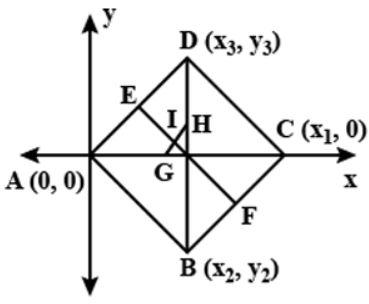Courses
Courses for Kids
Free study material
Free LIVE classes
MoreLIVE
Join Vedantu’s FREE Mastercalss

# Prove that the lines joining the middle points of opposite sides of a quadrilateral and the line joining the middle points of its diagonals meet in a point and bisect one another.Verified
364.5k+ views
Hint: - Here, we made a quadrilateral whose one diagonal is x axis. And then suppose the coordinate of the corner of the quadrilateral with respect to x axis and y axis. And then go through the bisector formula of coordinates.Let ABCD be the quadrilateral such that diagonal AC is along x axis suppose the coordinates A, B, C and D be $(0,0),({x_2},{y_2})({x_1},0)$and $({x_3},{y_3})$ respectively.
E and F are the mid points of sides AD and BC respectively, G and H are the midpoint of diagonals AC and BD. And the point of intersection of EF and GH is I.
Coordinates of E are $\left( {\dfrac{{0 + {x_3}}}{2},\dfrac{{0 + {y_3}}}{2}} \right) = \left( {\dfrac{{{x_3}}}{2},\dfrac{{{y_3}}}{2}} \right)$
Coordinates of Fare$\left( {\dfrac{{{x_1} + {x_2}}}{2},\dfrac{{0 + {y_2}}}{2}} \right) = \left( {\dfrac{{{x_1} + {x_2}}}{2},\dfrac{{{y_2}}}{2}} \right)$
Coordinates of midpoint of EF are $\left( {\dfrac{{\dfrac{{{x_3}}}{2} + \dfrac{{{x_1} + {x_2}}}{2}}}{2},\dfrac{{\dfrac{{{y_3}}}{2} + \dfrac{{{y_2}}}{2}}}{2}} \right) = \left( {\dfrac{{{x_1} + {x_2} + {x_3}}}{4},\dfrac{{{y_2} + {y_3}}}{4}} \right)$
G and H are the mid points of diagonal AC and BD respectively then

Coordinates of G are$\left( {\dfrac{{0 + {x_1}}}{2},\dfrac{{0 + 0}}{2}} \right) = \left( {\dfrac{{{x_1}}}{2},0} \right)$
Coordinates of H are$\left( {\dfrac{{{x_2} + {x_3}}}{2},\dfrac{{{y_2} + {y_3}}}{2}} \right)$
Coordinates of midpoint of GH are $\left( {\dfrac{{\dfrac{{{x_1}}}{2} + \dfrac{{{x_2} + {x_3}}}{2}}}{2},\dfrac{{\dfrac{{{y_2}}}{2} + \dfrac{{{y_2} + {y_3}}}{2}}}{2}} \right) = \left( {\dfrac{{{x_1} + {x_2} + {x_3}}}{4},\dfrac{{{y_2} + {y_3}}}{4}} \right)$
As you can see, midpoints of both EF and GH are the same. So, EF and GH meet and bisect each other.
Hence, proved.

Note:-Whenever we face such types of questions first of all make the diagram by the statement given by the question, then use the section formula to find the midpoint of a line. If the two points have the same value then it must coincide.
Last updated date: 27th Sep 2023
Total views: 364.5k
Views today: 6.64k